# Coordinate Geometry: Concepts, Formulas & Examples Notes | Study Quantitative Aptitude (Quant) - CAT

## CAT: Coordinate Geometry: Concepts, Formulas & Examples Notes | Study Quantitative Aptitude (Quant) - CAT

The document Coordinate Geometry: Concepts, Formulas & Examples Notes | Study Quantitative Aptitude (Quant) - CAT is a part of the CAT Course Quantitative Aptitude (Quant).
All you need of CAT at this link: CAT

Introduction

• A coordinate graph is a rectangular grid with two number lines called axes. The x-axis is the horizontal number line and the y-axis is the vertical number line.
• The axes intersect at the origin which is the point (0,0).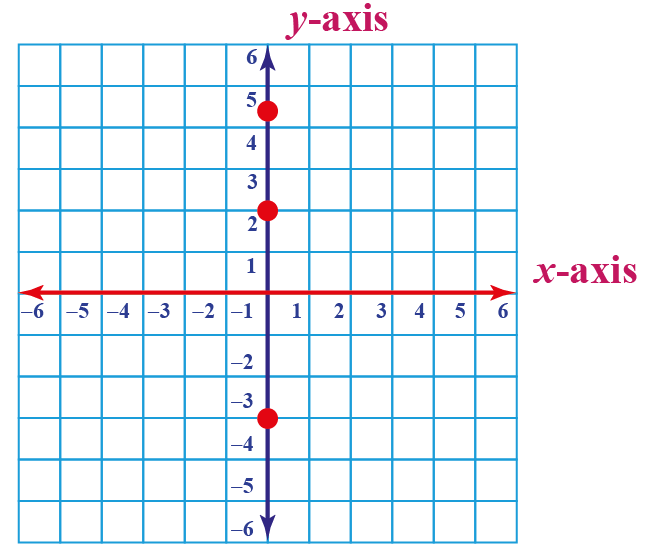Coordinate Geometry Formulas

• Now, Let us have a look at some formulas for coordinate geometry. We will use the below picture as a reference for the formulas.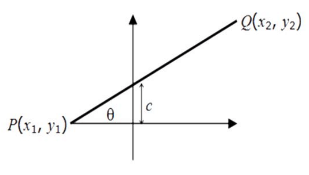•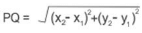• Slope of PQ = m =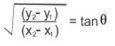• Equation of PQ is as below: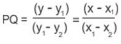or y = mx + c
• The product of the slopes of two perpendicular lines is –1.
• The slopes of two parallel lines are always equal. If m1 and m2 are slopes of two parallel lines, then m1=m2.
• The distance between the points (x1, y1) and (x2, y2) is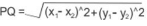• If point P(x, y) divides the segment AB, where A  (x1, y1) and B   (x2, y2), internally in the ratio m: n, then,
x= (mx2 + nx1)/(m+n)  and  y= (my2 + ny1)/(m+n)
• If P is the midpoint then,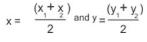• If G (x, y) is the centroid of triangle ABC, A  (x1, y1), B   (x2, y2), C   (x3, y3), then,
x = (x1 + x+ x3)/3 and y = (y1 + y2 + y3)/3
• If I (x, y) is the in-center of triangle ABC, A   (x1, y1), B   (x2, y2), C   (x3, y3), then,
•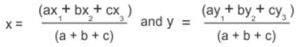where a, b and c are the lengths of the BC, AC and AB respectively.
• The equation of a straight line is y = mx + c, where m is the slope and c is the y-intercept (tan   = m, where   is the angle that the line makes with the positive X-axis).
• If two intersecting lines have slopes m1 and m2 then the angle between two lines θ will be tan θ = (m1−m2) / (1+m1m2)
• The length of perpendicular from a point (x1 ,y1 ) on the line AX+BY+C = 0 is
P = (Ax1+By1+C) / (A2+B2)

Equations of a Line

• General equation of a line Ax + By = C
• Slope intercept form y = mx + c (c is y intercept)
• Point-slope form y – y1 = m (x – x1) (m is the slope of the line)
• Intercept form x / a + y / b = 1 (where a and b are x and y intercepts respectively)
• Two point form:  (y−y1) / (y2−y1) = (x−x1) / (x2−x1)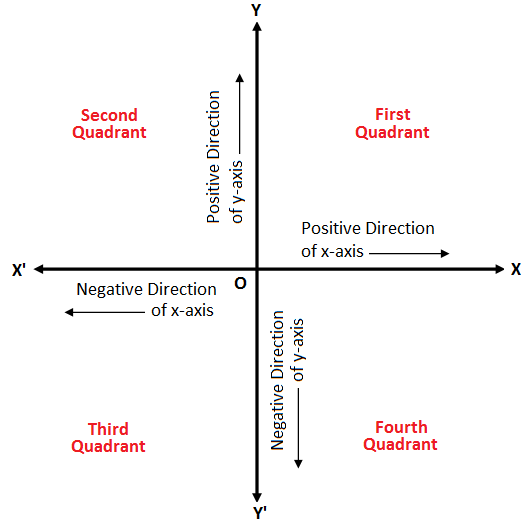• Quadrant I ⇒ X is Positive Y is Positive
• Quadrant II ⇒ X is Negative Y is Positive
• Quadrant III ⇒ X is Negative Y is Negative
• Quadrant IV ⇒ X is Positive Y is Negative

Try yourself:Angles between 180 ° and 270 ° lies in:

Solved Examples

Example 1: What is the distance between the points A (3,8) and B(-2,-7) ?
a) 5√2
b) 5
c) 5√10
d) 10√2

• The distance between 2 points (x1, y1) and (x2, y2) is given as
• Sqrt ((x2-x1)2 + (y2-y1)2)
• Hence, required distance = sqrt((-2-3)2 + (-7-8)2) = 5√10

Example 2: The points of intersection of three lines 2X + 3Y – 5=0 and 5X – 7Y + 2=0 and 9X – 5Y – 4 = 0
a) Form a triangle
b) Are on lines perpendicular to each other
c) Are on lines parallel to each other
d) Are coincident

To solve the question above, we should remember the properties of the lines for being parallel, perpendicular or intersecting:

• Two lines are parallel to each other if their slopes are equal
• Two lines are perpendicular if the product of slopes is -1
• Lines are coincident if they at least have one point which satisfies all the equation.
• The three lines can be expressed in the y=mx + c format as:
Y = (5/3) – (2X/3),   Y = (5X/7) + (2/7)   , Y = (9X/5) – (4/5)
• Therefore, the slopes of the three lines are -2/3, 5/7, 9/5 and their Y intercepts are 5/3, 2/7 and 4/5 respectively.
• We see above that the product of slopes of none of the lines is -1. Thus, lines are not perpendicular to each other.
• Also, slopes of the no two lines is same. Thus, lines are not parallel to each other.
• Solving the first two equations we get X=1 and Y = 1. If we substitute (1,1) in the third equation Y=(9X/5 – 4/5), we find that it also satisfies the equation. This suggests that the three lines intersect at a common point and hence coincident.

Example 3: The area of the triangle whose vertices are (a + 1, a + 1), (a, a) and (a+2, a) is
a) a3
b) 1
c) 2a
d) 21/2

• Let a = 0, Thus the three vertices of the triangle becomes (1, 1) (0, 0) and (2, 0)
• If we look at the below figure, Area = ½ * base * height = ½ * 2 * 1 = 1
• Imp: The main point to note here is that area will be independent of a.

Example 4: Consider a triangle drawn on the X – Y plane with its three vertices of (41,0) , (0,41) and (0,0), each vertex being represented by its (X,Y) coordinates. The number of points with integer coordinates inside the triangle (excluding all the points on the boundary) is:
a) 12
b) 8
c) 6
d) 4

• Equation of the line will be of the form => x + y = 41.
• Now, we know that if the x,y coordinates of a point are integer, the sum will also be an integer
X + y = k (k, a variable)
• As per the question we need to exclude all the values lying on the boundary of triangle, k can take all values from 1 to 40 only. K = 0 is rejected as at k =0 will give the point at A which is also not allowed.
• With K = 40, x + y = 40; this will be satisfied by points (1, 39), (2, 38), (3,37) …… (38, 2), (39, 1). That is a total of 39 points
• Similarly x + y = 38, will be satisfied by 37 points.
X + Y = 37, will be satisfied by 36 points
X + Y = 3 will be satisfied by 2 points
X + Y = 2 will be satisfied by 1 point
X + Y = 1 will not be satisfied by any point
• So, the total number of all such points is: 39 + 38 + 37 + 36 + ……………………. + 3 + 2 + 1 = n(n+1)/2 points =  (39*40) / 2 = 780 points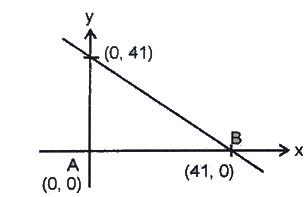Example 5: Two lines P and Q intersect at point (3, 2) in the x-y plane. The slope of line P is 45 degrees and line Q is parallel to the X axis. What is the area (in sq. units) of the triangle formed by P, Q and a line perpendicular to P passing through point (5, 4) ?
a) 12
b) 8
c) 6
d) 4

• Let us look at the image below: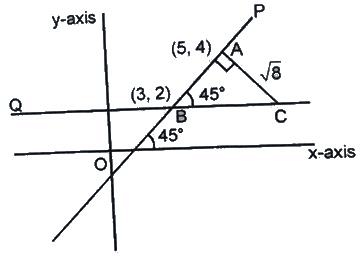• As slope of line P is 45 degree. Therefore, ∠ABC = 45 degree
• In triangle ABC, length of AB = SQRT [(5-3)+ (4-2)2] = 22 units
• Therefore, length of line AC = 22 units (Since ABC is an isosceles triangle. Thus AB = AC)
• Thus, required area = ½ * 22 * 22 = 4 sq. units

Example 6: The line 3 Y = x is the radius of the circle. It meets the circle o=centered at origin O at point M (3, 1). If PQ is the tangent to the circle at M as shown, find the length of the PQ.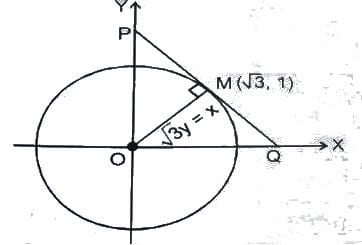a) (5/2)3 units
b) 3 3 units
c) 23 units
d) 8/3 units

• PQ is perpendicular to line Y = X / 3 (Since, radius of a circle is perpendicular to the tangent of the circle)
• Therefore, slope of PQ = -1 / (1/ 3) = – 3 (Since, product of slopes of line perpendicular to each other is -1)
• Therefore, Let equation of the line PQ be y = – 3x + c
• Now at the point M, when x = 3, y = 1
• Putting the above values of x and y in the above equation, we get c = 4 The equation of the line becomes, Y = – 3x + 4
• Thus, by using the above equation, we get:
• Coordinates of point P = (0, 4) and coordinates of point Q = (4/3, 0) (Putting x = 0 in above equation, we find value of P and putting Y = 0 in above equation, we find value of Q)
• Hence PQ = sqrt [(4/3) + 42] = 8/3 units.
The document Coordinate Geometry: Concepts, Formulas & Examples Notes | Study Quantitative Aptitude (Quant) - CAT is a part of the CAT Course Quantitative Aptitude (Quant).
All you need of CAT at this link: CATUse Code STAYHOME200 and get INR 200 additional OFF

## Quantitative Aptitude (Quant)

155 videos|129 docs|131 tests

### How to Prepare for CAT

Read our guide to prepare for CAT which is created by Toppers & the best Teachers

Track your progress, build streaks, highlight & save important lessons and more!

,

,

,

,

,

,

,

,

,

,

,

,

,

,

,

,

,

,

,

,

,

,

,

,

;